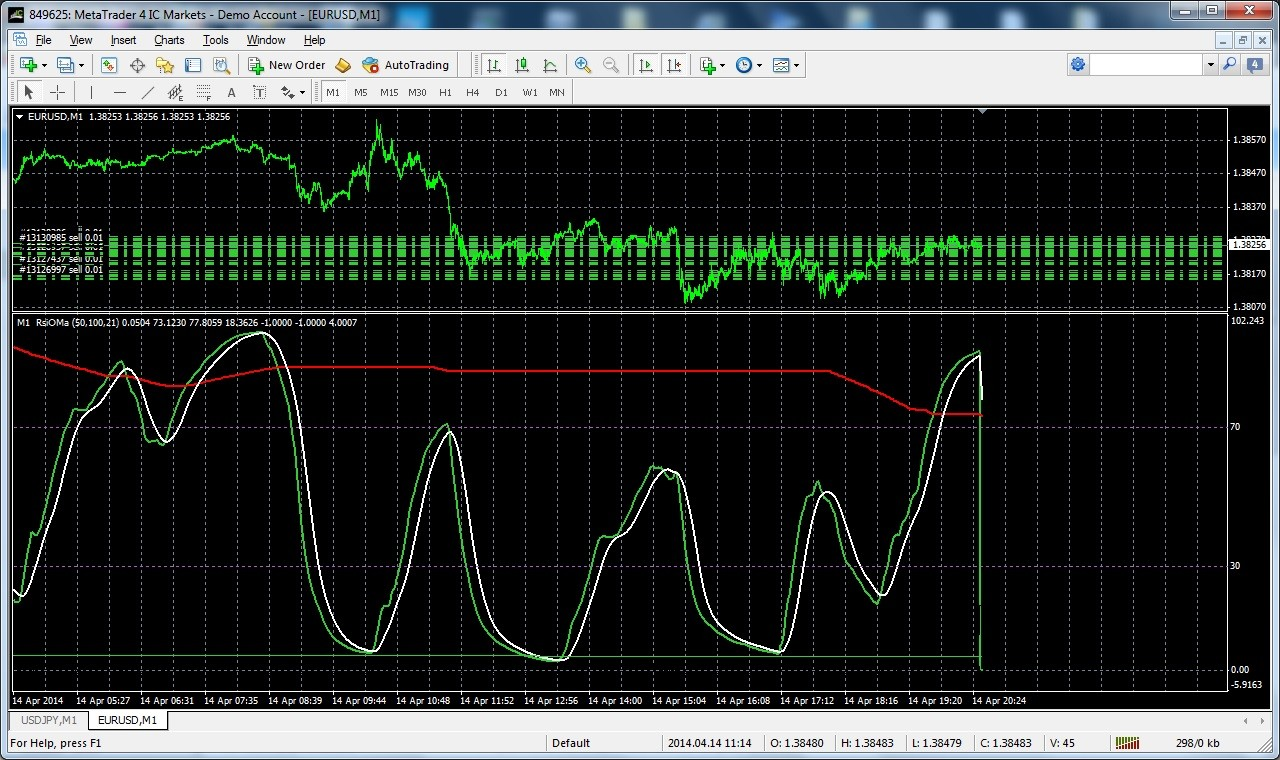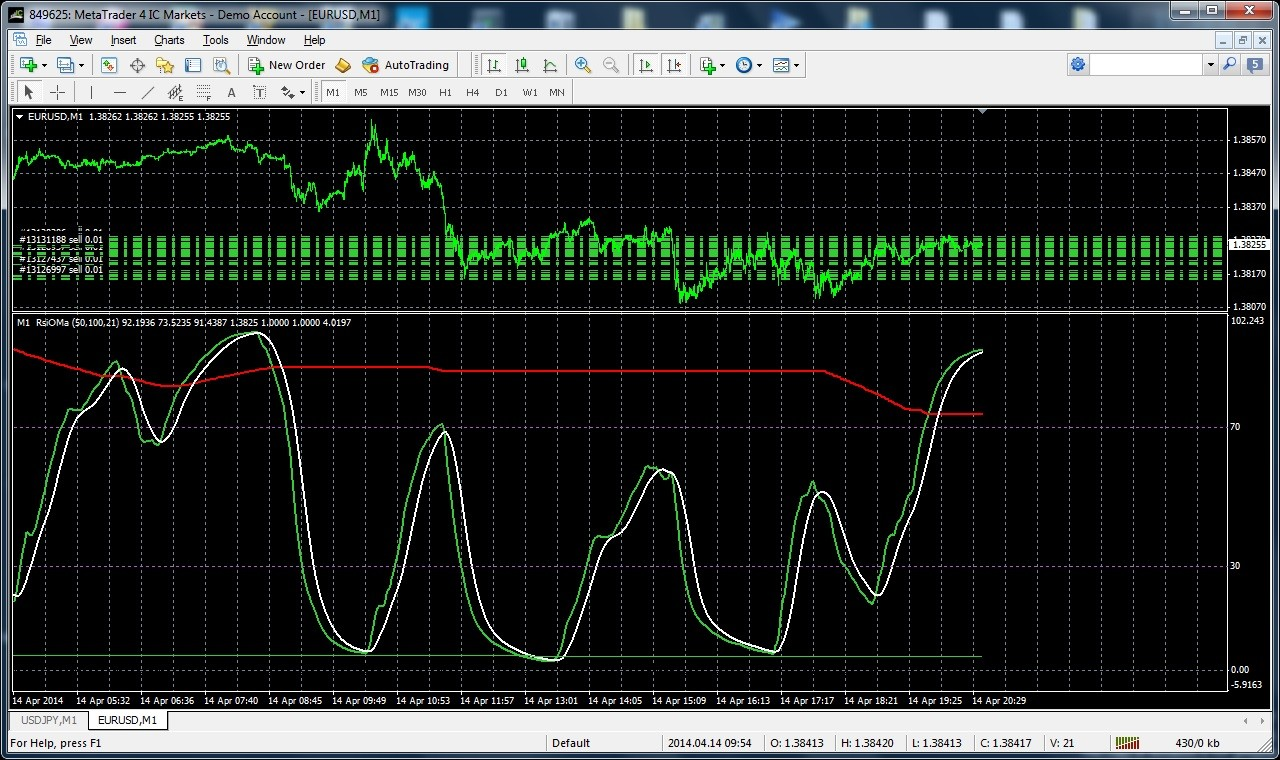# RSI of Moving Average - page 35155883

fajst_k:
this is a classic trick with 'Cyprus company' to be EU company. Otherwise they would have to pay import EU taxes in case of distributing MT4 with EU brokers.....

But that also could be an explanation why we are forced to use "new" metatrader 4 : if they were hit by the Cyprus accounts shave, then they are broke. Come to think of it, the whole story about the new metatrader 4 came somewhere after the Cyprus thing happened. Not sure. Someone correct me if I am wrong (about the timeline)1232

It was bout the same time (the Cyprus)1232

Checked it : Cyprus "shave" was at the end of March. Already in June they started to announce changes in metatrader 4 (it took them 1 month to come up with the solution for the money they lost there. And now we are forced to use this only because they were laundering money through Cyprus298

Here is a set of basic averages that are all working correctly

When I replaced the iMA() and iMAOnArray() calls to calls to these functions, in the case I had those errors, all became OK. Might help, who knows

//------------------------------------------------------------------

//

//------------------------------------------------------------------

//

//

//

//

//

#define _maWorkBufferx1 1 //

#define _maWorkBufferx2 2 // to be multiplied with the ttal numeber

#define _maWorkBufferx3 3 // of averages instances that will be used

#define _maWorkBufferx5 5 //

double iCustomMa(int mode, double price, double length, int i, int instanceNo=0)

{

int r = Bars-i-1;

switch (mode)

{

case 1 : return(iEma(price,length,r,instanceNo));

case 2 : return(iSmma(price,length,r,instanceNo));

case 3 : return(iLwma(price,length,r,instanceNo));

default : return(iSma(price,(int)length,r,instanceNo));

}

}

//

//

//

//

//

double workSma[][_maWorkBufferx2];

double iSma(double price, int period, int r, int instanceNo=0)

{

if (ArrayRange(workSma,0)!= Bars) ArrayResize(workSma,Bars); int k = period; instanceNo *= 2;

//

//

//

//

//

workSma[r] = price;

if (r>=period)

workSma[r] = workSma[r-1]+workSma[r]-workSma[r-period];

else { workSma[r] = 0; for(k=0; k=0; k++) workSma[r] += workSma[r-k]; }

return(workSma[r]/k);

}

//

//

//

//

//

double workEma[][_maWorkBufferx1];

double iEma(double price, double period, int r, int instanceNo=0)

{

if (ArrayRange(workEma,0)!= Bars) ArrayResize(workEma,Bars);

//

//

//

//

//

double alpha = 2.0 / (1.0+period);

if (r>0)

workEma[r] = workEma[r-1]+alpha*(price-workEma[r-1]);

else workEma[r] = price;

return(workEma[r]);

}

//

//

//

//

//

double workSmma[][_maWorkBufferx1];

double iSmma(double price, double period, int r, int instanceNo=0)

{

if (ArrayRange(workSmma,0)!= Bars) ArrayResize(workSmma,Bars);

//

//

//

//

//

if (r<period)

workSmma[r] = price;

else workSmma[r] = workSmma[r-1]+(price-workSmma[r-1])/period;

return(workSmma[r]);

}

//

//

//

//

//

double workLwma[][_maWorkBufferx1];

double iLwma(double price, double period, int r, int instanceNo=0)

{

if (ArrayRange(workLwma,0)!= Bars) ArrayResize(workLwma,Bars);

//

//

//

//

//

workLwma[r] = price;

double sumw = period;

double sum = period*price;

for(int k=1; k=0; k++)

{

double weight = period-k;

sumw += weight;

sum += weight*workLwma[r-k];

}

return(sum/sumw);

}

I'm using your code as a replacement iMA and iMAOnArray like this

if (!detrend)for (i=limit; i >= 0; i--) mab = iCustomMa(Before_Mode,Close,Period_Before,i,0);

than sometimes (not always) I'm getting this on the screen (see vertical green rsi plot)Files:
notok.jpg 380 kb155883

fajst_k:
I'm using your code as a replacement iMA and iMAOnArray like this

if (!detrend)for (i=limit; i >= 0; i--) mab = iCustomMa(Before_Mode,Close,Period_Before,i,0);

than sometimes (not always) I'm getting this on the screen (see vertical green rsi plot)Are you using averaging more than once in the code?298

But when I use just iMA than is ok

if (!detrend)for (i=limit; i >= 0; i--) mab = iMA(NULL,0,Period_Before,0,Before_Mode,0,i);

I think its related to ticks and real time work as vertical rsi green line occurs only in case of RT streaming.

Any idea ??

KrzysztofFiles:
ok.jpg 378 kb298

Are you using averaging more than once in the code?

Yes, later I'm using it again155883

fajst_k:
Yes, later I'm using it again

The multiply the constants in this part :

#define _maWorkBufferx1 1

#define _maWorkBufferx2 2

#define _maWorkBufferx3 3

#define _maWorkBufferx5 5

(the 1,2,3 and 5) with the number of instances you are using it and use different instance number for each new instance (the last parameter) and then try it out. It has to work correctly298

The multiply the constants in this part :
#define _maWorkBufferx1 1

#define _maWorkBufferx2 2

#define _maWorkBufferx3 3

#define _maWorkBufferx5 5
(the 1,2,3 and 5) with the number of instances you are using it and use different instance number for each new instance (the last parameter) and then try it out. It has to work correctly

and now is OK.

Thanks, Krzysztof155883

fajst_k:
and now is OK. Thanks, Krzysztof

GoodOne more mql trap avoided. Happy coding and trading